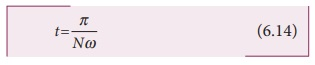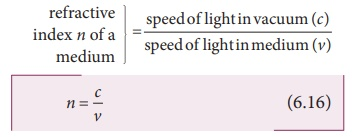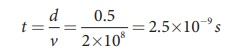Home | | Physics 12th Std | Speed of Light

# Speed of Light

Light travels with the highest speed in vacuum. The speed of light in vacuum is denoted as c .

SPEED OF LIGHT

Light travels with the highest speed in vacuum. The speed of light in vacuum is denoted as c and its value is, c = 3×108 ms-1. It is a very high value. Several attempts were made by scientists to determine the speed of light. The earliest attempt was made by a French scientist Hippolyte Fizeau (1819– 1896). That paved way for the other scientists too to determine the speed of light.

## Fizeau’s method to determine speed of light

Apparatus: The apparatus used by Fizeau for determining speed of light in air is shown in Figure 6.13. The light from the source S was first allowed to fall on a partially silvered glass plate G kept at an angle of 45º to the incident light from the source. The light then was allowed to pass through a rotating toothed-wheel with N teeth and N cuts of equal widths whose speed of rotation could be varied through an external mechanism (not shown in the Figure). The light passing through one cut in the wheel will get reflected by a mirror M kept at a long distance d, about 8 km from the toothed wheel. If the toothed wheel was not rotating, the reflected light from the mirror would again pass through the same cut and reach the eyes of the observer through the partially silvered glass plate.

Working: The angular speed of rotation of the toothed wheel was increased from zero to a value ω until light passing through one cut would completely be blocked by the adjacent tooth. This is ensured by the disappearance of light while looking through the partially silvered glass plate.

Expression for speed of light: The speed of light in air v is equal to the ratio of the distance the light travelled from the toothed wheel to the mirror and back 2d to the time taken t.The distance d is a known value from the arrangement. The time taken t for the light to travel the distance to and fro is calculated from the angular speed ω of the toothed wheel.

The angular speed ω of the toothed wheel when the light disappeared for the first time is,Here, θ is the angle between the tooth and the slot which is rotated by the toothed wheel within that time t.Substituting for θ in the equation 6.13. for ω,Rewriting the above equation for t,Substituting t from equation (6.14) in equation (6.12),After rearranging,Fizeau had some difficulty to visually estimate the minimum intensity of the light when blocked by the adjacent tooth, and his value for speed of light was very close to the actual value. Later on, with the same idea of Fizeau and with much sophisticated instruments, the speed of light in air was determined as, v = 2.99792×108 m s–1.

After the disappearance of light for the first time while increasing the speed of rotation of the toothed-wheel from zero to ω, on further increase of speed of rotation of the wheel to 2ω, the light would appear again due to the passing of reflected light through the next slot. So, for every odd value of ω, light will disappear (stopped by tooth) and for every even value of ω light will appear (allowed by slot).

## Speed of light through different media

Different transparent media like glass, water etc. were introduced in the path of light by scientists like Foucault (1819– 1868) and Michelson (1852–1931) to find the speed of light in different media. Even evacuated glass tubes were also introduced in the path of light to find the speed of light in vacuum. It was found that light travels with lesser speed in any medium than its speed in vacuum. The speed of light in vacuum was determined as, c = 3×108 m s-1. We could notice that the speed of light in vacuum and in air are almost the same.

## Refractive index

Refractive index of a transparent medium is defined as the ratio of speed of light in vacuum (or air) to the speed of light in that medium.Refractive index of a transparent medium gives an idea about the speed of light in that medium.

### EXAMPLE 6.5

One type of transparent glass has refractive index 1.5. What is the speed of light through this glass?

SolutionLight travels with a speed of 2 × 108 ms-1 through this glass.

Refractive index does not have unit. The smallest value of refractive index is for vacuum, which is 1. For any other medium refractive index is greater than 1. Refractive index is also called as optical density of the medium. Higher the refractive index of a medium, greater is its optical density and speed of light through the medium is lesser and vice versa. [Note: optical density should not be confused with mass density of the material of the medium. They two are different entities]. The Table 6.2 shows the refractive index of different transparent media.

### Table 6.2 Refractive index of different mediaMedia : Refractive index

Vacuum: 1.00

Air: 1.0003

Carbon dioxide gas: 1.0005

Ice: 1.31

Pure water: 1.33

Ethyl alcohol: 1.36

Quartz: 1.46

Vegetable oil: 1.47

Olive oil: 1.48

Acrylic: 1.49

Table salt: 1.51

Glass: 1.52

Sapphire: 1.77

Zircon: 1.92

Qubic zirconia: 2.16

Diamond: 2.42

Gallium phosphide: 3.50

## Optical path

Optical path of a medium is defined as the distance d' light travels in vacuum in the same time it travels a distance d in the medium.

Let us consider a medium of refractive index n and thickness d. Light travels with a speed v through the medium in a time t. Then we can write,

v = d/t ; rewrittenas, t = d/v

In the same time, light can cover a greater distance d' in vacuum as it travels with greater speed c in vacuum as shown in Figure 6.14. Then we have,c= d′/t ; rewritten as, t = d′/c

As the time taken in both the cases is the same, we can equate the time t as,rewritten for the optical path d' as, d′ = c/v  d

As, c/v = n; The optical path d' is,As n is always greater than 1, the optical path d' of the medium is always greater than d.

### EXAMPLE 6.6

Light travels from air in to glass slab of thickness 50 cm and refractive index 1.5.

(i) What is the speed of light in glass?

(ii) What is the time taken by the light to travel through the glass slab?

(iii) What is the optical path of the glass slab?

### Solution

Given, thickness of glass slab, d = 50 cm = 0.5 m, refractive index, n = 1.5

refractive index, n = c/v

speed of light in glass is,Time taken by light to travel through glass slab is,Optical path,

d′ = nd = 1.5×0.5 = 0.75 m = 75 cm

Light would have travelled 25 cm more (75 cm – 50 cm) in vacuum by the same time had there not been a glass slab.

Tags : Optics | Physics , 12th Physics : UNIT 6 : Ray Optics
Study Material, Lecturing Notes, Assignment, Reference, Wiki description explanation, brief detail
12th Physics : UNIT 6 : Ray Optics : Speed of Light | Optics | Physics